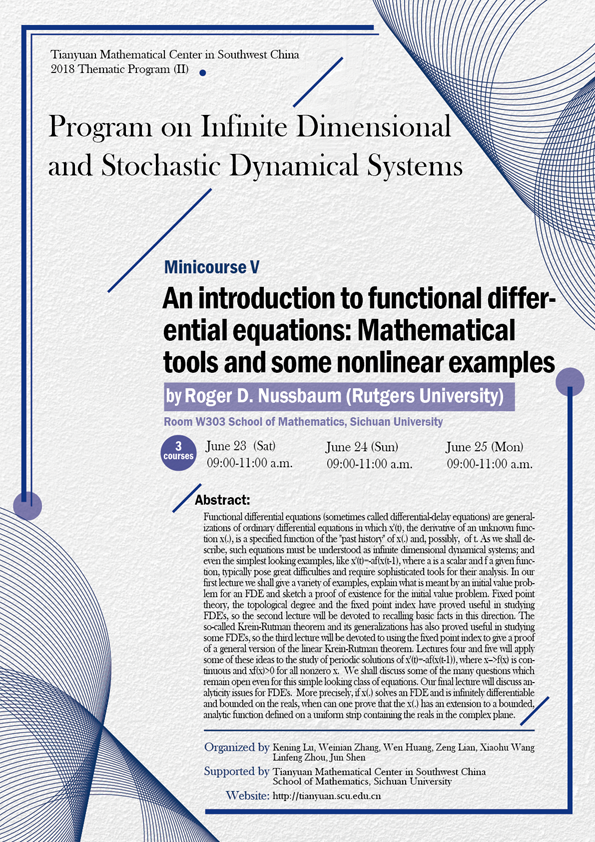2018 Thematic Program (II)

### An introduction to functional differential equations:Mathematical tools and some nonlinear examples

#### W303  School of Mathematics, Sichuan University#### SPEAKER

Roger D. Nussbaum (Rutgers University)

#### ABSTRCT

Functional differential equations (sometimes called differential-delay equations) are generalizations of ordinary differential equations in which x'(t), the derivative of an unknown function x(.), is a specified function of the "past history" of x(.) and, possibly, of t. As we shall describe, such equations must be understood as infinite dimensional dynamical systems; and even the simplest looking examples, like x'(t)=-af(x(t-1), where a is a scalar and f a given function, typically pose great difficulties and require sophisticated tools for their analysis. In our first lecture we shall give a variety of examples, explain what is meant by an initial value problem for an FDE and sketch a proof of existence for the initial value problem. Fixed point theory, the topological degree and the fixed point index have proved useful in studying FDE's, so the second lecture will be devoted to recalling basic facts in this direction. The so-called Krein-Rutman theorem and its generalizations has also proved useful in studying some FDE's, so the third lecture will be devoted to using the fixed point index to give a proof of a general version of the linear Krein-Rutman theorem. Lectures four and five will apply some of these ideas to the study of periodic solutions of x'(t)=-af(x(t-1)), where x-->f(x) is continuous and xf(x)>0 for all nonzero x. We shall discuss some of the many questions which remain open even for this simple looking class of equations. Our final lecture will discuss analyticity issues for FDE's. More precisely, if x(.) solves an FDE and is infinitely differentiable and bounded on the reals, when can one prove that the x(.) has an extension to a bounded, analytic function defined on a uniform strip containing the reals in the complex plane.

#### ORGANIZERS

Kening Lu (Sichuan University)

Weinian Zhang (Sichuan University)

Wen Huang (Sichuan University)

Zeng Lian (Sichuan University)

Xiaohu Wang (Sichuan University)

Linfeng Zhou (Sichuan University)

Jun Shen (Sichuan University)

#### SUPPORTED BY

Tianyuan Mathematical Center in Southwest China

School of Mathematics, Sichuan University